Courses

# Matrices & Determinants IIT JAM Notes | EduRev

## IIT JAM: Matrices & Determinants IIT JAM Notes | EduRev

The document Matrices & Determinants IIT JAM Notes | EduRev is a part of the IIT JAM Course IIT JAM Mathematics.
All you need of IIT JAM at this link: IIT JAM

Matrices

A matrix A over a field K or, simply, a matrix A (when K is implicit) is a rectangular array of scalars usually presented in the following form: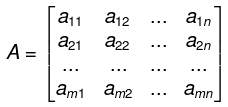The rows of such a matrix A are the m horizontal lists of scalars:
(a11, a12, ..., a1n), (a21, a22, ..., a2n), ..., (am1, am2, ..., amn)
and the columns of A are the n vertical lists of scalars: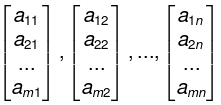Note that the element aij, called the ij-entry or ij-element, appears in now i and column j.
We frequently denote such a matrix by simply writing A = [aij].

A matrix with m rows and n columns is called an m by n matrix, written m * n. The pair of numbers m and n is called the size of the matrix. Two matrices A and B are equal, written A = B, if they have the same size and if corresponding elements are equal. Thus the equality of two m * n matrices is equivalent to a system of mn equalities, one for each corresponding pair of elements.

A matrix with only one row is called a row matrix or row vector and a matrix with only one column is called a column matrix or column vector. A matrix whose entries are all zero is called a zero matrix and will usually be denoted by 0.

Matrices whose entries are all real numbers are called real matrices and are said to be matrices over R. Analogously, matrices whose entries are all complex numbers are called complex matrices and are said to be matrices over ℂ.

Example:
(a) The rectangular array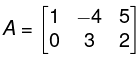is a 2 x 3 matrix. Its rows are (1, - 4 , 5)  and (0,3, -2), and its columns are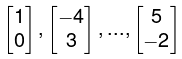(b) The 2 x 4 zero matrix is the matrix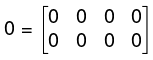.

Algebra of matrix

➤ Matrix Addition and Scalar Multiplication
Let A = [ aij and B = [bij] be two matrices with the same size , say m * n matrices. The sum of A and B, written A + B, is the matrix obtained by adding corresponding elements from A and B. That is,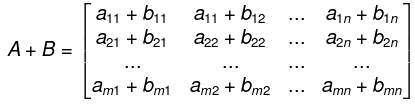The product of the matrix A by a scalar k, written k • A or simply kA, is the matrix obtained by multiplying each element of A by k. That is,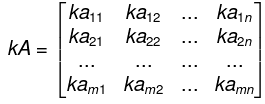Observe that A + B and kA are also m * n matrices. We also define
-A= (-1) A and A - B = A + (-B)
The matrix - A is called the negative of the matrix A, and the matrix A - B is called the difference of A and B. The sum of matrices with different sizes is not defined.

Example: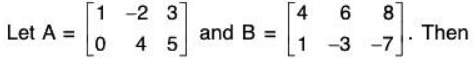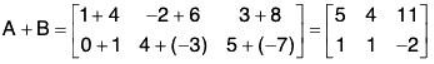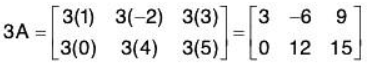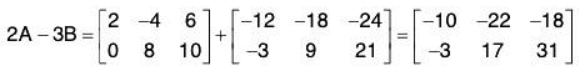The matrix 2A - 3B is called a linear combination of A and B.
Basic properties of matrices under the operations of matrix addition and scalar multiplication follows.

Theorem.: Consider any matrices A, B, C (with the same size) and any scalars k and k’. Then:
(i) (A + B) + C = A + (B + C ),
(ii) A + 0 = 0 + A = A,
(iii) A + (- A) = (- A) + A = 0,
(iv) A + B = B + A,
(v) k(A + B) = kA + kB,
(vi) (k + k’)A = kA + k’A,
(vii) (k k’)A = k (k’A),
(viii) 1· A = A.

➤ Matrix Multiplication
The product of matrices A and B, written AB, is somewhat complicated. For this reason, we first begin with a special case.
The product AB of a row matrix A = [aj and a column matrix B = [b] with the same number of elements is defined to be the scalar (or 1 * 1 matrix) obtained by multiplying corresponding entries and adding; that is,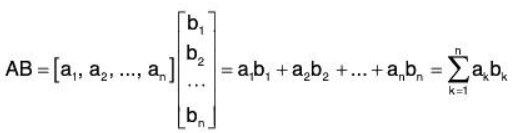We emphasize that AB is a scalar (or a 1 x 1 matrix). The product AB is not defined when A and B have different numbers of elements.
Example: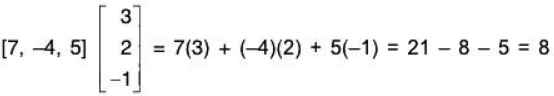➤ Definition
Suppose A = [aik] and B = [bkj] are matrices such that the number of columns of A is equal to the number of rows of B; say, A is an m * p matrix and B is a p * n matrix. Then the product AB is the m x n matrix whose ij-entry is obtained by multiplying the ith row of A by the jth column of B. That is,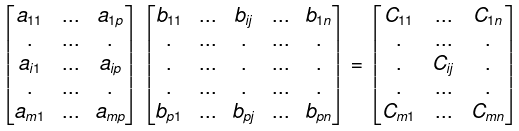where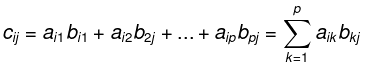The product AB is not defined if A is an m * p matrix and B is a q * n matrix, where p * q. Theorem: Let A, B, C be matrices. Then, whenever the products and sums are defined:
(i) (AB)C = A(BC) (associative law),
(ii) A(B + C) = AB + AC (left distributive law),
(iii) (B + C)A = BA + CA (right distributive law),
(iv) k(AB) = (kA)B = A(kB), where k is a scalar.
We note that OA = 0 and BO = 0, where 0 is the zero matrix.

➤ Transpose of a Matrix

The transpose of a matrix A, written AT, is the matrix obtained by writing the columns of A, in order, as rows. For example,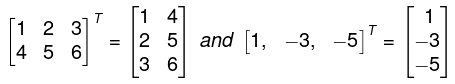In other words, if A = [aij] is an m * n matrix, then AT = [bij] is the n * m matrix where bij = aji. Observe that the transpose of a row vector is a column vector. Similarly, the transpose of a column vector is a row vector.
The next theorem lists basis properties of the transpose operation.

Theorem: Let A and B be matrices and let k be a scalar. Then, whenever the sum and product are defined:
(i) (A + B)T = AT + BT,
(ii) (AT)T = A,
(iii) (kA)T = kAT,
(iv) (AB)T = BTAT.

➤ Square Matrices
A square matrix is a matrix with the same number of rows as columns. An n * n square matrix is said to be of order n and is sometimes called an n-square matrix.

Example: The following are square matrices of order 3.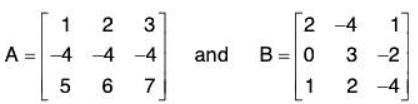The following are also matrices of order 3: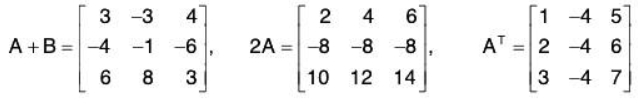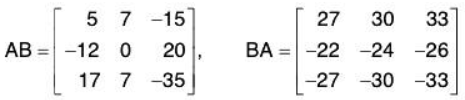Diagonal and Trace
Let A = [ aij] be an n-square matrix. The diagonal or main diagonal of A consists of the elements with the same subscripts, that is,
a11, a22, a33, ... ann
The trace of A, written tr(A), is the sum of the diagonal elements. Namely,
tr(A) = a11+ a22+ a33+ ...+ ann
The following theorem applies.
Theorem: Suppose A = [aij] and B = [bij] are n-square matrices and k is a scalar. Then:
(i) tr(A + B) = tr(A) + tr(B),
(ii) tr(kA) = k tr(A),
(iii) tr(AT) = tr(A),
(iv) tr(AB) = tr(BA).

Example:
Let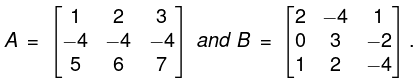Then
diagonal of A = {1, -4, 7} and tr(A) = 1 - 4 + 7 = 4
diagonal of B = {2, 3, -4} and tr(B) = 2 + 3 - 4 = 1
Moreover,
tr(A + B ) = 3 - 1 + 3 = 5.
tr(2A) = 2 - 8 + 14 = 8.
tr(AT) = 1 -4 + 7 = 4
tr(AB) = 5 + 0 - 35 = -30,
tr(BA) = 27 - 24 - 33 = -30
As expected from Theorem,
tr(A + B) = tr(A) + tr(B),
tr(AT) = tr(A), tr(2A) = 2 tr(A)
Furthermore, although AB * BA, the trace are equal.

Identity Matrix, Scalar Matrices
The n-square identity or unit matrix, denoted by ln, or simply I, is the n-square matrix with 1’s on the diagonal and 0's elsewhere. The identity matrix I is similar to the scalar 1 in that, for any n-square matrix A,
Al = IA = A
More generally, if B is an m x n matrix, then Bln = lmB = B.
For any scalar k, the matrix kl that contains k’s on the diagonal and 0's elsewhere is called the scalar matrix corresponding to the scalar k. Observe that
(kl)A = k(IA) = kA
That is, multiplying a matrix A by the scalar matrix kl is equivalent to multiplying A by the scalar k.

Example : The following are the identity matrices of orders 3 and 4 and the corresponding scalar matrices for k = 5;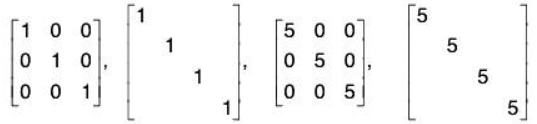Remark 1: It is common practice to omit blocks or patterns of 0's when there is no ambiguity, as in the above second and fourth matrices.
Remark 2: The Kronecker delta function δij is defined by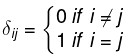Thus the identity matrix may be defined by I = [δij]

➤ Power of Matrices, Polynomials in Matrices
Let A be an n-square matrix over a field K. Powers of A are defined as follows:
A2 = AA,  A3 = A2A,  ... , An+1 = An A, ....,  and A= I
Polynomials in the matrix A are also defined. Specifically, for any polynomial
f(x) = a0 + a1x + a2x2 +...+ anxn
where the ai are scalars in K, f(A) is defined to be the following matrix:
f(x) = a0I + a1A + a2A2 +...+ anAn

[Note that f(A) is obtained from f(x) by substituting the matrix A for the variable x and substituting the scalar matrix a0l for the scalar a0.] If f(A) is the zero matrix, then A is called a zero or root of f(x).

Example: Suppose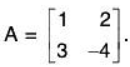Then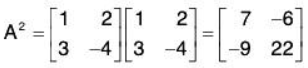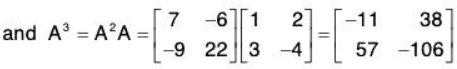Suppose f(x) = 2x2 - 3x + 5 and g(x) = x2 + 3x - 10. Then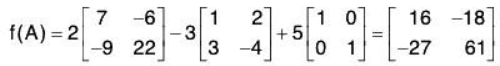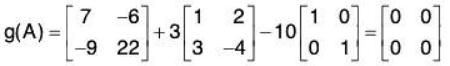Thus A is a zero of the polynomial g(x).

Types of Matrices

1. Row Matrix: If a matrix has only one row and any number of columns, is called a Row matrix, or a row vector ; e.g. [2 7 3 9] is a row matrix.
2. Column Matrix: A matrix having one column and any number of rows is a called a column matrix is a column vector, example: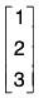is a column matrix
3. Null Matrix of Zero Matrix: Any matrix in which all the elements are zeros is called a Zero matrix or Null matrix, i.e.,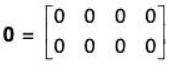. In general, a m * n matrix in which each element is zero, is called anull matrix of the type m * n and is denoted by Om*n.
4. Square Matrix: A matrix in which the number of rows is equal to the number of columns is called a square matrix i.e. A = (aij)m*n is a square matrix if and only if m=n.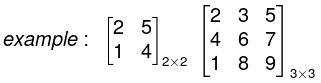are square matrices.
5. Diagonal Matrix: A square matrix is a called a diagonal matrix if all its non-diagonal elements are zero i.e. in general a matrix A = (aij)mxn is called a diagonal matrix if aij = 0 for i ≠ j;
for example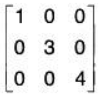is a diagonal matrix of order 3 * 3 i.e. 3 alone.
6. Unit or Identity Matrix: A square matrix is called a unit matrix if all the diagonal elements are unity and non-diagonal elements are zero.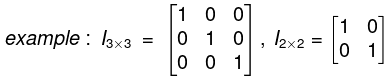are Identity matrices of order 3 x 3 and 2 * 2 respectively.
7. Symmetric Matrix: A square matrix is called symmetric if for all values of i and j, aij = aji . ⇒ A’ = A i.e. transpose of the matrix A = A ;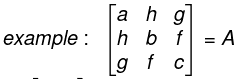is a symmetric matrix of order 3 * 3 .
8. Skew Symmetric Matrix: A square matrix is called skew symmetric matrix if aij = -aji, for all values of i and j and all its diagonal elements are zero. ⇒ A’ = - A i.e. transpose of the matrix A = -A;
example.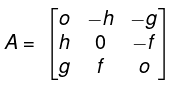is a skew symmetric matrix of order 3 x 3.
9. Triangular Matrix: A square matrix, in which all its elements below the leading diagonal are zero, is called an up per triangular matrix while square matrix, all of whose elements above the leading diagonal are zero, is called a lower triangular matrix.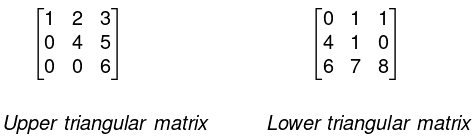10. Transpose of Matrix: If in a given matrix, we interchange the rows and the corresponding columns, the new matrix obtained is called the transpose of the matrix A and is denoted by A or At or AT.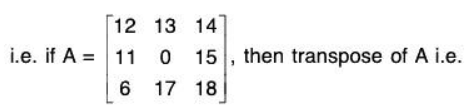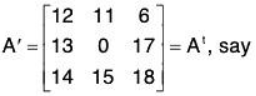11. Orthogonal Matrix: A square matrix A is called an orthogonal matrix if the product of the matrix A with its transpose matrix A’ is an identity matrix.
i.e. AA’ = I.
12. Conjugate of a Matrix: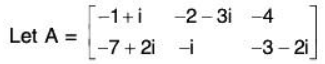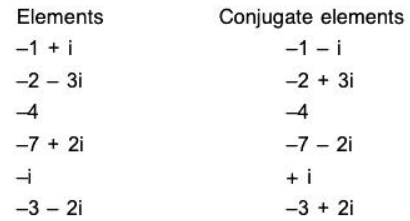∴ Conjugate of matrix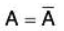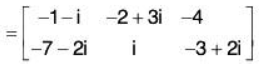13. Matrix Aθ : Transpose of the conjugate of a matrix A is denoted by Aθwe have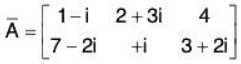and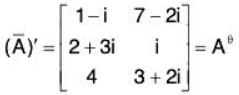14. Unitary Matrix: A square matrix A is said to be unitary, if we have AθA =1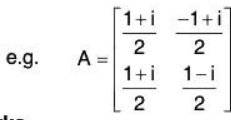Remarks
• A real matrix is unitary if and only if it is orthogonal.
• If P is unitary so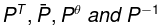are also unitary.
• If P and Q are unitary so PQ is unitary multiple.
• If P is unitary, then I P I  is of unit modulus.
• Any two Eigen vectors corresponding to the distinct Eigen values of a unitary matrix are orthogonal.
• If P is orthogonal so PT and P-1 are also orthogonal.
• If P and Q are orthogonal so PQ is also orthogonal.
• If P is orthogonal, then IPI = ± 1.
15. Hermitian Matrix: A square matrix A = (aij) is said to be Hermitian Matrix if every i- jth element of A is equal to conjugate  complex of j - ith element of A, or in order words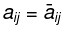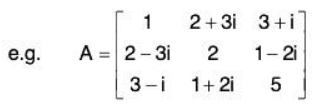The necessary and sufficient condition for the matrix A to be Hermitian is that A = Aθ i.e. conjugate transpose of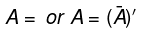.
16. Skew Hermitian Matrix: A square A = (aij) is said to be a Skew Hermitian matrix if every j-ith element of A is equal to negative conjugate complex of j-ith element of A; i.e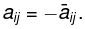All the elements of the principal diagonal are of the form: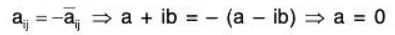Hence aij is purely imaginary i.e. aij = 0 + ib or aij = 0.
i.e. all the diagonal element of a Skew Hermitian Matrix are either zeros or pure imaginary.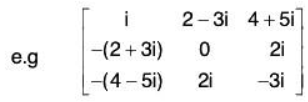Thus the necessary and sufficient condition for a matrix A to be Skew Hermitian is that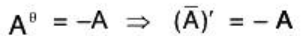Properties
• If A is a Skew-Hermitian matrix, then kA is also Skew-Hermitian for any real number k.
• If A and B are Skew-Hermitian matrices of same order, then λ1A +λ2B is also Skew Hermitian for real number λ1,λ2.
• If A and B are Hermitian matrices of same order, then AB-BA is Skew-Hermitian.
• If A is any square matrix, then A - A* is Skew-Hermitian matrices.
• Every square matrix can be uniquely represented as the sum of a Hermitian and a Skew Hermitian matrices.
• If A is a skew-Hermitian (Hermitian) matrix, then iA is a Hermitian matrix, then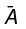also Skew-Hermitian (Hermitian).
17. Idempotent Matrix: A matrix such that A2 = A is called Idempotent Matrix.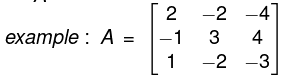18. Periodic Matrix: A matrix A is said to be a Periodic Matrix if Ak+1 = A, where k is a +ve integer. If k is the least +ve integer for which Ak +1 = A then k is said to be period of A. If we choose k = 1, then A2 = A and we call it to be an idempotent matrix.
19. Invoiutory Matrix: A matrix A is said to an involutory matrix if A2 = I (unit matrix). Since A2 = I always, we conclude that Unit matrix is always involutory.
20. Nilpotent Matrix: A matrix is said to be a Nilpotent matrix if Ak = 0 (null matrix) where k is +ve integer; if however k is the least +ve integer of which Ak = 0, then k is called as the index of the nilpotent matrix.
The matrix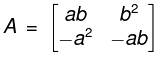is nilpotent and its index is 2.
21. Submatrix: A matrix obtained from a given matrix, say A = (aij)mxn by deleting some rows or columns or both is called a submatrix of A.
22. Principal diagonal: The elements a11, a33, ..., amn of a square matrix of order m*m are called diagonal elements and the diagonal containing these elements is called the principal diagonal.  In a square matrix (aij)m x m, aij is the diagonal element if i = j.

➤ Equal Matrices
Two matrices are equal if

1. They are of the same order.
2. The elements in the corresponding positions are equal.

Thus if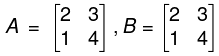Here A = B.

In general if A = (aij)m x d and B = (bjj)m x n are matrices each of order m * n and aij = bij for all i and j then A = B.

Note:

1. An orthogonal matrix P is said to be proper if IPI = 1, improper if IPI = - 1.
Clearly, P-1 is proper of both improper, the PQ is proper if PQ is improper then one of P, Q is improper.
2. A square matrix is unitary if and only if its columns (rows) form an orthogonal set of unit vectors.
3. A square matrix is orthogonal if and only if its columns (rows) form an orthogonal set of unit vectors.
4. Let x, be any unit n-vector. Then there exists a unitary matrix U having x1 as its first column.

Rank of Matrix

Minor of a Matrix
Let A be a matrix square or rectangular, from it delete all rows leaving a certain t rows and all columns leaving a certain t columns. Now if t > 1 then the elements that are left, constitute a square matrix of order t and the determinant of this matrix is called a minor of A of order t.
A single element of A may be consider as minor of order 1.

➤ Rank of a Matrix
A number r is said to be the rank of a matrix A if it possesses the following two properties.

1. there is at least one minor of A of order r which does not vanish.
2. every minor of A of order (r + 1) or high vanish. Ail minors of order greater than r will vanish if all minors of order (r + 1) are zero.

Rank of a matrix is the order of the highest non-vanishing minor of the matrix. From the above definition we have the following two useful results.

1. The rank of matrix A is ≥ r, if there is at least one minor of A of order r which does not vanish.
2. The rank of matrix A is ≤ r if all the minors of A of order (r + 1) or higher vanish.

The rank of a matrix whose minors of order n are all zero is < n. We assign the rank n to the matrix which has at least one non-zero minor of order n; n being the order of any highest minor of the matrix, e.g. the rank of every onward non-singular matrix is n.
Again rank of every non-zero matrix is ≥ 1, we assign the rank zero to every zero matrix.
We shall denote the rank of matrix A by the symbol ρ(A).

Example. Find the rank of the matrix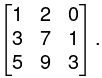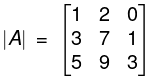= 1(21 - 9) - 2 (9 - 5) + 0 (27 - 35)
= 12 - 8 = 4 ≠ 0.
ρ(A) = 3

➤ Rank of a Matrix (by elementary transformations of a matrix)
Consider a matrix A = (aij)m x n then its rank can be easily calculated by applying elementary transformations given below:

1. Interchange any two rows (i.e. Rij) or columns (Cij).
2. Multiplication of the elements of a row (or a column) say Ri(or Ci) by a non zero number k is denoted by k Ri(or kCi)
3. The addition to the elements of ith row (or column) the corresponding elements of any other row say jth row (or column) multiplied by a non zero number k is denoted by Rij(k) or Cij(k). Any elementary operation is called a row transformation (or a column transformation) according as it operates on row (or column). In easy form we use the following notations:
• Ri ↔ Rj (interchange of ith and jth row) (Ci ↔ Cj (interchange of ith and jth column)
• Ri ↔ k Ri(Ci → k Ci) means multiply ith row (or column) by k ≠ 0.
• R.↔ Ri + kRj (Ci → Ci + kC) means multiply each element of jth row (or column) by non zero number k and add it to the corresponding elements of ith row (column).

The rank of a matrix does not alter by applying elementary row transformation (or column transformations).

Rank of a Matrix A = (aij)m*n by Reducing it to Normal Form.
Every non zero matrix [say A = (aij)m*n] of rank r, by a sequence of elementary row (or column) transformations be reduced to the forms: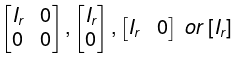where lr is a r*r unit matrix o f order r and 0 denotes null matrix of any order. These forms are called as normal form or canonical form of the matrix A. The order or of the unit matrix lr is called the rank of the matrix A.

The rank of a matrix does not alter by pre-multiplication or post-multiplication with a non singular matrix.

➤ Echelon Form of a Matrix
A matrix A = (aij)m*n is said to be in ECHELON FORM, if

1. all the non zero rows, if any, precede the zero rows,
2. The number of zeros preceding the first non zero element in a row is less than that the number of such zeros in the succeeding (or next) row,
3. The first non zero element in every row is unity.
Example: the matrix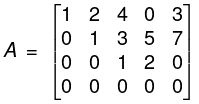is in echelon form.
Since in A,
• The zero rows precede the zero row.
• The number of zero preceding the first non zero element in rows R2, R3, R4 are 1, 2 and 5 which are in ascending order.
• The first non zero element in each row is 1.

NOTE: When a matrix is converted in Echelon form, then the number of non zero rows of the matrix is the rank of the matrix A.

Example. Determine the rank of the matrix, by E-transformations.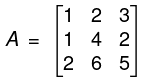Now,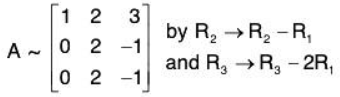Now applying the elementary transformations.
R3→ R3 - R2, we have
or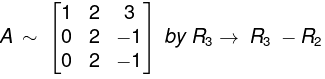Here third order minor viz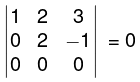(vanishes) while the second order minor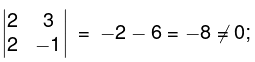so the rank of the matrix is 2.

➤ Elementary matrix
A matrix obtained by the application of any one of the elementary row (or column) operation to the identity matrix is called an elementary row (or column) matrix.

The following notations are used for the elementary row matrices.
(i) Eij Elementary row matrix obtained by the operation Rij.
(ii) Ei(k) Elementary row matrix obtained by the operation kRi.
(iii) Eij(k) Elementary row matrix obtained by the operation Ri + kRj.
(iv) E’ij(k) transpose of elementary matrix Eij(k), which can also be obtained by the operation Cij(k).

Inverse of a Matrix

➤ Definition
Let A be a square matrix. If there exists a matrix B, such that AB = I = BA, Where I is an unit matrix, then B is called the inverse of A and is denoted by A-1 and matrix A is called non-singular matrix. The matrix A is called non-singular matrix if such matrix B does not exist.

Note: The matrix B = A-1 will also be a square matrix of the same order as A.

➤ Theorems on Inverse of a matrix
Theorem. Every invertible matrix possesses a unique inverse.

Theorem. The inverse of the product of matrices of the same type is the product of the inverses of the matrices in reverse order i.e.
(AB)-1 = B-1 A-1, (ABC)-1 = C-1 B-1 A-1 and (A-1 B-1)-1 = BA.

Theorem. The operations of a transpose and inverting are commutative, i.e. (A’)-1 = (A-1)’ where A is a m x n non-singular matrix, i.e. det A≠ 0.

Theorem. If a sequence of elementary operations can reduce a non-singular matrix A of order n to an identity matrix, then the sequence of the same elementary operations will reduce the identity matrix ln to inverse of A.
i.e. If (Er.Er_1,  E2 . E1)A = ln
then (Er.Er_1 .. E2.E1) ln = A-1.

This is also known as Gauss-Jorden reduction method for finding inverse of a matrix.

Theorem. The inverse of the conjugate transpose of a matrix A (order m * n) is equal to the conjugate transpose of the matrix inverse to A, i.e. ( Aθ)-1 = (A-1)θ.

Method of finding inverse of a non-singular matrix by elementary transformations.
The method is that we take A and I (same order) and apply the row operations on both successively elementary transformations till A become I. When A reduces to I, I reduces to A-1.

Example. Find the inverse of the matrix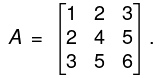Write A = IA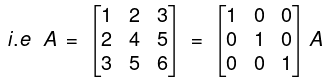By R2 - 2R1, R3 - 3R1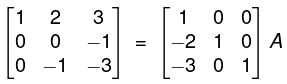By R2 + R3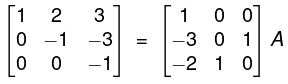By R, + 3R3, R2 - 3R3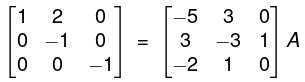By R2 + 2R2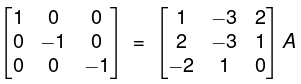By - R2, - R3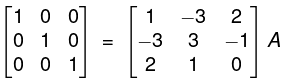⇒ I = BA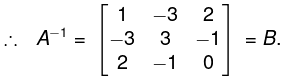➤ Inverse of a Matrix (another form)

The inverse of A is given by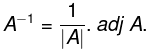The necessary and sufficient condition for the existence of the inverse of a square matrix A is that IAI ≠ 0, i.e. matrix should be non-singular.

Properties of inverse matrix:
If A and B are invertible matrices of the same order, then

1. ( A-1)-1 = A.
2. (AT)-1 = (A-1)T
3. (AB)-1 = B-1A-1
4. (Ak)-1 = (A-1)k , k ∈
6.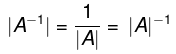7.  A = diag (a1,a2....an) ⇒ A-1 = diag (a1-1a2-2..., an-1)
8. A is symmetric matrix ⇒ A-1 is also symmetric matrix.
9. A is diagonal matrix, IAI ≠ 0 ⇒ A-1 is also triangular matrix.
10. A is scalar matrix ⇒ A-1 is also a scalar matrix.
11. A is triangular matrix, IAI ≠ 0 ⇒ A-1 is also triangular matrix.
12. Every invertible matrix possesses a unique inverse.
13. Cancellation law with respect to multiplication

If A is a non-singular matrix i.e., if IAI ≠ 0, then A-1 exists and
AB = AC ⇒ A-1 (AB) = A-1(AC)
⇒ (A-1A)B = (A-1A)C
⇒ IB = IC ⇒ B = C
∴ AB = AC ⇒ B = C = ⇔ IAI ≠ 0.

Determinants

Consider the following simultaneous equations
a11x + a12y = k1,
a21x + a22y = k2
these equations have the solution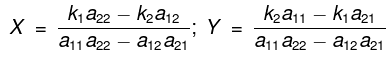provided the expansion a11a22 - a12a21 ≠ 0. This expression a11a22 - a12a21 is represented by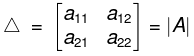and is called a determinant of second order. The number a11,a12,a21,a22 are called elements of the determinant. The value of the determinant is equal to the product of the elements along the principal diagonal minus the product of the off-diagonal elements.
Similarly a symbol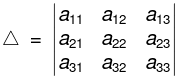is called a determinant of order 3 and its value is given by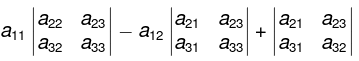= a11 (a22a33 - a32a23) - a12(a21a33 - a31a23 ) + a13(a21a32 - a31a22)   ...(1)

Rule
a11 (determinant obtained by removing the row and column intersecting at a11). - a12 (determinant obtained by removing the row and column intersecting at a12) + a13 (determinant obtained by removing the row and column intersecting at a13).
This is called expansion of the determinant along the first row.

Note: In a determinant of order 3 there are 3 rows and 3 columns and its value can be found by expanding it along any of its rows of along any of its columns. In these expansions the element a., is multiplied by (-1)i+j to fix the sign of aij.

➤ Properties of Determinants

1. The value of the determinant does not change by changing rows into columns and columns into rows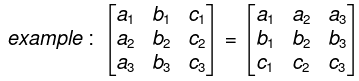2. If any two adjacent rows (or columns) of a determinant are interchanged, the value of the determinant is multiplied by -1.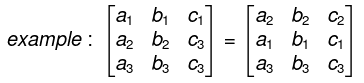Note: In case any row (or column) of a determinant A be passed over n rows (or columns) then the resulting determinant is (-1)n Δ.
(fourth row has passed over three parallel rows)
3. If any two rows or two columns of a determinant are identical then the determinant are identical then the determinant vanishes.
4. If all the elements of any row (or any column) of a determinant are multiplied by the same factor k then the value of new determinant is k times the value of the given determinant.
5. If in a determinant each element in any row (or column) consists of the sum of r terms, the determinant can be expressed as the sum of r determinants of the same order.
6. If to the elements of a row (or column) of a determinant are added m times the corresponding elements of another row (or column), the value of the determinant so obtained is equal to the value of the original determinant.

General rule. One should always try to bring in as many zeros as possible in any row (column) and then expand the determinant with respect to that row (column)
Thus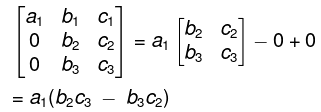➤ Minors and cofactors
Minors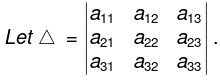If we omit the row and the column passing through the element aij, then the second order determinant so obtained is called the minor of the element aij and is denote by Mij.
Therefore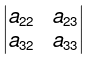is minor of a11.

Similarly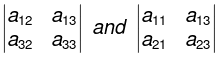are the minors of the elements a21 and a32 respectively.

Cofactors: The minor Mij multiplied by (-1)i+j is called the cofactor of the element aij. Cofactor of aij =Aij = (-1)i+j Aij.

➤ Reciprocal Determinant
If in a given determinant each element is replaced by its cofactor, then the determinant so formed is called reciprocal or inverse determinant of the given determinant. If the original determinants is A then its reciprocal determinant is denoted by Δ’.
If A is a determinant of order n and A’ be its reciprocal determinant then Δ = Δn-1.

Factor theorem. If the elements of a determinant Δ are functions of x and two parallel lines become identical when x = a, then x - a is a factor of Δ.

➤ Properties of Determinants

1. When at least one row (or column) of a matrix is a linear combination of the other rows (or columns) the determinant is zero.
2. The determinant of an upper triangular or lower triangular matrix is the product of the main diagonal entries.
3. The determinant of the product of two square matrices is the product of the individual determinants:
|A.B| = IA|.|B|
One immediate application is to matrix powers:
IAI2 = IAI.IAI
and more generally IAnl = IAIn for integer n.
4. The determinant of the transpose of a matrix is the same as that of the original matrix:
IATI = IAI.
The document Matrices & Determinants IIT JAM Notes | EduRev is a part of the IIT JAM Course IIT JAM Mathematics.
All you need of IIT JAM at this link: IIT JAMUse Code STAYHOME200 and get INR 200 additional OFF Use Coupon Code
All Tests, Videos & Notes of IIT JAM: IIT JAM## IIT JAM Mathematics

106 videos|27 docs|30 tests

Track your progress, build streaks, highlight & save important lessons and more!

,

,

,

,

,

,

,

,

,

,

,

,

,

,

,

,

,

,

,

,

,

;